All submissions of the EM system will be redirected to Online Manuscript Submission System. Authors are requested to submit articles directly to Online Manuscript Submission System of respective journal.

# How, When, Where and Why Life Began in Our Universe

Paul TE Cusack*

Eng, Dule 1641 Sandy Point Rd, Saint John Nb, Canada

*Corresponding Author:
Paul TE Cusack
Eng, Dule 1641 Sandy Point Rd
E-mail: [email protected]

Received date: 10/07/2016; Accepted date: 24/07/2016; Published date: 28/07/2016

Visit for more related articles at Research & Reviews: Journal of Statistics and Mathematical Sciences

## Abstract

This paper examines the mathematical conditions necessary for life to begin. It shows, by calculation, where life would begin in the universe, and what energy levels were necessary. Life Began 3.5 BYA in the region surrounding the Earth.

#### Keywords

Origin of Life, Creation, Cosmology.

#### Introduction

Based on my paper, Astro-Theology, Cusack’s Universe, I hereby propose the solution as to how life began in our universe and where. The foundation is Genesis, but the methods include Energy calculation necessary of the chemical reactions to support and sustain simple life forms [1,2]. What follows are the calculations that lead to life in our universe, nearby.

c^2=E/t=6.7320

1-E/t=0.8515=Ehat

Ehat+E^2-1=0

c^2+0.8515-1=0

Ehat=1-c^2

Ehat=1/t=7.98~8

Ehat=1/t

Ehat is the energy to allow life to be created.

1 qusackian, qu = 45 degrees or Pi/4 radsEhat=E-E^2

E^2-E+Ehat=0FMa

=dM/dt (dv/dt)

dM/dt(d2s/dt2)

F = Es/(c^2t)

26.667=0.84(0.1334)/(3^2)(t)

t=0.0125

E-1/tE=Ehat

E-E/t=Ehat

tc^2=E

0.8415/0.0125)=6.7320

1-E/t=0.8515

I will show that life was inevitable and occurred in the time from as be expected. The sin curve and the cosine curve are the two poles: one is life; the other is no life. Where they meet is where the energy density is just right to cause life to firm. There are 25 pages of mathematical calculations. Life began 666,000,000 years ago. I assume the simplest form of life; the amoeba is the first life form to form on earth. There probably is life on other planets where there is water. Intelligent life may have been a fluke on earth. But it is part of god’s plan for humans to know right from wrong. That is when the Jewish year 3761 BC. began with Adam and Eve (Figure 1).[1,2].

Figure 1: Genesis account of life creation, Christian Community Bible, Claireton Press, Phillipines.

Genesis Text

In the beginning, when God began to create the heavens and the earth, the earth had no form and was void (No Mass or Matter - Just Energy); darkness was over the deep and the Spirit of God hovered over the waters (Primordial Waters: No Water No Life).

God said, “Let there be light” (Sun Energy: Necessary for Life); and there was light. God saw that the light was good and he separated the light from the darkness. God called the light ‘Day’ and the darkness ‘Night’. There was evening and there was morning: the first day.

God said, “Let there be a firm ceiling between the waters and let it separate waters from waters” (Atmospheric Oxygen). So God made the ceiling and separated the waters below it from the waters above it. And so it was. God called the firm ceiling ‘Sky’. There was evening and there was morning: the second day.

God said, “Let the waters below the sky be gathered together in one place (Oceans) and let dry land appear.”(Pangaea) And so it was. God called the dry land ‘Earth’, and the waters gathered together he called ‘Seas’. God saw that it was good.

God said, “Let the earth produce vegetation, seed-bearing plants, fruittrees bearing fruit with seed, each according to its kind, upon the earth.” i.e., Life. And so it was. The earth produced vegetation: plants bearing seed according to their kind and trees producing fruit which has seed, according to their kind. God saw that it was good. There was evening and there was morning: the third day. (A Day Is Like A Week: 7 Billion Years/22 by=3.182=1/Pi).

God said, “Let there be lights in the ceiling of the sky to separate day from night and to serve as signs for the seasons, days and years; and let these lights in the sky shine above the earth.” And so it was. God therefore made two great lights, the greater light to govern the day and the smaller light to govern the night; and God made the stars as well.

Delta Ehat/dt=0.10/0.1387=0.7210

Cone

V'=Pi R h

=dEhat/dt=V=Pi (26.667)h

delta h = 0.0086062

Vol=Pi R^2h

Vol=0.86=Pi (26.667)^2(0.861)/3

ho=0.0324

delta h/ho=0.0086/0.0324=0.2656

=15.22 degrees

e^0.1522=1/0.858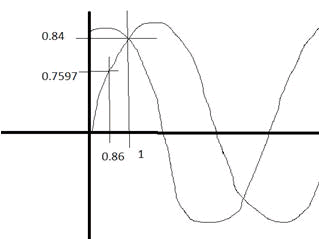Life Ehat-Universe Ehat=0.1016

Ehat-UEhat=0.1016

1-(Ehat-U Ehat)=9.84

Ehat-0.202=0.1016+0.202

Ehat=0.3036

Delta t=0.1387=s

Delta Ehat=0.86-0.76

=0.10

0.10*Pi=0.314

1/Ehat=31.8 Hz = freq

1/Ehat=freq

1/dE=1/dt

dt=dE=1

dE/dt=1

Delta Ehat=dE

delta Ehat/dt=dE/dt=1

0.1/0.1387=0.7210

x^2-x-1=0

2x-1=0

2x-1=dE/dt=1

x=1=t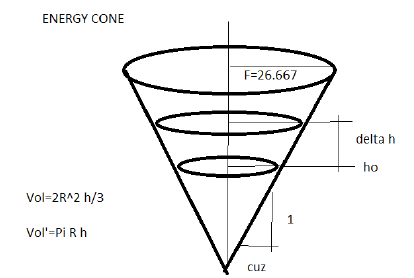Vol =PiR^2h/3

Vol'=Pi R h

dVol /dt=dE/dt=Pi R h

R=F=26.667

Pi(26.667)(h=e

Vol=Pi R^2h

=Pi*26.667*(0.0324/3

=0.2413

1-0.2413=0.7587

1-i=0

1-0.618=0.3856

E^2-E+Ehat=0

E=0.0247

sin E=cos E=EhatY=e^-tcos (2Pi t)

2Y=2e^-tcos (2Pi t)

t=1

2Y=2e^-1)(cos (2Pi t)

2Y=2e^-t

2Y=0.7358

Y=2.718=e

Y=Y'

Life was Inevitable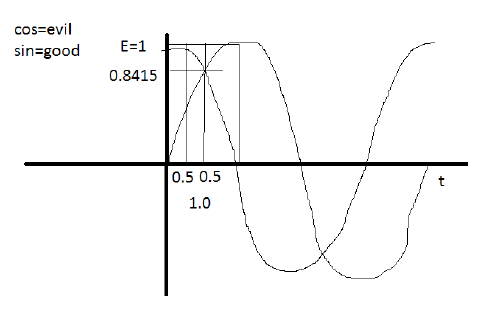E?2-E+Ehat=0

E?2-E+0.8415=0

E(E-1)+0.8415=0

E(E-1)=-0.8415

E=-0.8415/(1-E)

x=0.8415/(1-x)

x^2-x+0.8415=0

x=0.0247, 0.9753

Y=0.0247

1/Y=40.4=2(0.202)=2Y

2Y^2=1

Y=0.707=1/sqrt 2

Y=sin 45 =cos 45

Ehat=1/t

Ehat=/sec 1/Pi)(0.8515)=27.0777

Work=W

W*Ehat=freq

Ehat=freq/W

Ehat=1

Ehat=1/t=1/1=1

31.8 Hz /W

1/Pi*Pi =1

"converging on 1" ==> Golden mean

t?2-t-1=0

t=1.618=x

But t=1

1=1.618 (sqrt -1)

1ésqrt (-1)=1.618

1.618 (sqrt (-1)-1=0

1-i=0

y=mx +b

i=b+mx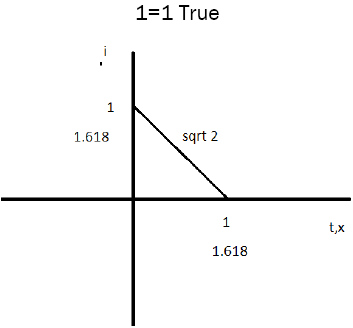Rate of a Reaction

Amoeba

670,000,000,000/666,000,000=100 Reactions per year.

Rate 1=2.71 reactions /day

Ehat = cuz=0.4233/reaction

Rate x Energy =2.71 x 0.4233=1/1506=Energy Expenditure

0.869~0.868=t

W=Energy consumption

Ehat=1/t Ehat=0.866

Endothermic Reaction

5 N 1P 9C 6 O+delta Ehat ==> Amoeba

137 KJ/bond x 32 bonds +0.869==> 670 x 10^9

t=0.2625 15.03 degrees e^0.1503=1.16

=0.8604

Energy Density

Vol of Ellipsoid =4/3 Pi abc

=4/3Pi (66)(24)(3)

=19905

Ehat=).8515 *Pi

=0.001344

~s

Y=e^-t* cos (2Pi t)

=0.1281*0.7441)=0.0955

s=0.5044

~1/2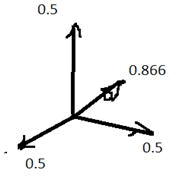Summary of the Conditions Necessary for Life

t=6.66 x 10-^9 ya

Ehat=cuz

E rho=3/4

s=0.5

Vector s=0.866 60 degrees

M=0.0946

E rho=0.75=3/4=c/4

c=4E rho

c^2=16E rho

E=Mc^2

Ehat=M (16 Ehat -rho)^2

Ehat=M*9

=c=3

Ehat=M*9

0.8515=M*9

M=0.0946

Eigen value / Eigen vector=0.5/0.866=0.5774 (Hebrew Year 2014 AD)t=1/ cuz = dE.

dt=1/cuz

t=Slope of energy function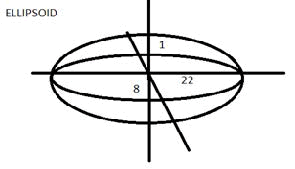2.22 Billion years ago, * 3 LY=6.66 billion years ago

6.7 Billion years ago

Ehat=0.8515

Ehat=1/t

0.8515=1Y=e^-t cos (2Piu t)

=e^6.66* (cos (2Pi 6.66)

=0.0010

Y/6.66=0.1502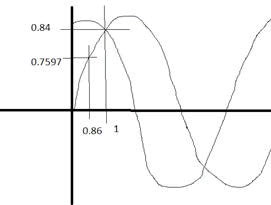delta h/ho=Ehat - UEhat)/UEhat) =1/Ehat

=0.2656

delta h /ho=Ehat-Uehat)/ UEhat=0.2656=1/Ehat

Ehat-UEhat=0.2656(UEhat)

X-Y=0.2656Y

Let Y=1

x-1=0.2656(1)

X-=0.2656+1

Ehat=x=1.2565

There are 32 atoms on the DNA chain.

32! =2.631×10^35

2.631/2.656=9,907 1.9.9070=0.1009

Life Began

t=0.0125

Ehat/t=1.2565/0.0125=0.1012

=Delta Ehat

Ehat/t=delta Ehat

Ehat/t=dEhat/dt=0.1012).

(0.1012)(Pi)=31.79~31.8 Hz

0.1012 x 22 =2.22 Billion years ago, life began after universe.

Ehat-UEhat=delta h=0.2656=15.22 degreese^15.22 degrees=1/0.858

1/EhatEhat-UEhat)/ UEhat=1/Ehat

x-y/y=1/x

x(x-y)=y

Let y=1

x-1=1/xx=1/x-1

Golden Mean

x=1.618

dE/dt=2x-1

2x-1=1

x=1=t

1/(6*2Pi)=1/12Pi=0.0265

2.65%

The universe is 22 Billion years old.

22BLY* 2.65%=5836

5836-5432=40.40=0.202 × 2=1/Y

E^2-E-Ehat=0

d=vit+1/2at^2

65.59=0+ ½ (2.9979)t^2

43.999=t^2

t^2/t=44/22=2

22 BLY *2.65%=1.749 ~ 1.75

1.75*2=3.5 billion years ago life began

t^2-t-1=E

E=4.4^2-4.4-1=0.14

=0.86

#### Conclusion

This confirms that, as expected, life began 3.5 billion years ago, the pre-requisite energy levels were just right at E=-sin 60.